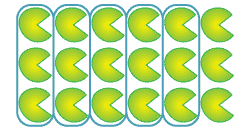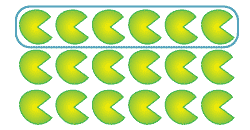#### Question 1

Select the correct fraction 'of ' 18 to match each diagram.

a   bDetailed description This diagram shows $$\dfrac{5}{6}$$ of 18 = 15 $$\dfrac{5}{18}$$ of 18 = 15 $$\dfrac{1}{15}$$ of 18 = 18Detailed description This diagram shows $$\dfrac{6}{12}$$ of 18 = 6 $$\dfrac{1}{3}$$ of 18 = 6 $$\dfrac{1}{6}$$ of 18 = 6

#### Question 2

Calculate:

a $$\dfrac{1}{2}$$ of 10 = $$\dfrac{3}{4}$$ of 20 = $$\dfrac{4}{6}$$ of 12 =